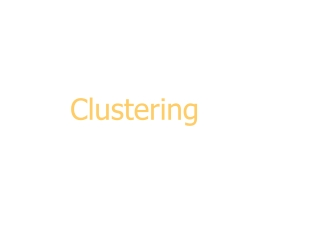DownloadDownload PresentationClustering

# Clustering

Download Presentation## Clustering

- - - - - - - - - - - - - - - - - - - - - - - - - - - E N D - - - - - - - - - - - - - - - - - - - - - - - - - - -
##### Presentation Transcript

1. Clustering

2. Introduction K-means clustering Hierarchical clustering: COBWEB Outline

3. Classification vs. Clustering Classification: Supervised learning: Learns a method for predicting the instance class from pre-labeled (classified) instances

4. Clustering Unsupervisedlearning: Finds “natural” grouping of instances given un-labeled data

5. Clustering Methods • Many different method and algorithms: • For numeric and/or symbolic data • Deterministic vs. probabilistic • Exclusive vs. overlapping • Hierarchical vs. flat • Top-down vs. bottom-up

6. d e a c j h k b f i g Clusters: exclusive vs. overlapping Simple 2-Drepresentation Non-overlapping Venn diagram Overlapping

7. Clustering Evaluation • Manual inspection • Benchmarking on existing labels • Cluster quality measures • distance measures • high similarity within a cluster, low across clusters

8. The distance function • Simplest case: one numeric attribute A • Distance(X,Y) = A(X) – A(Y) • Several numeric attributes: • Distance(X,Y) = Euclidean distance between X,Y • Nominal attributes: distance is set to 1 if values are different, 0 if they are equal • Are all attributes equally important? • Weighting the attributes might be necessary

9. Simple Clustering: K-means Works with numeric data only • Pick a number (K) of cluster centers (at random) • Assign every item to its nearest cluster center (e.g. using Euclidean distance) • Move each cluster center to the mean of its assigned items • Repeat steps 2,3 until convergence (change in cluster assignments less than a threshold)

10. Y k1 k2 k3 X K-means example, step 1 Pick 3 initial cluster centers (randomly)

11. Y k1 k2 k3 X K-means example, step 2 Assign each point to the closest cluster center

12. Y k1 k2 k2 k1 k3 k3 X K-means example, step3 Move each cluster center to the mean of each cluster

13. Y k1 k2 k3 X K-means example, step 4 Reassign points closest to a different new cluster center Q: Which points are reassigned?

14. Y k1 k3 k2 X K-means example, step 4 … A: three points with animation

15. Y k1 k3 k2 X K-means example, step 4b re-compute cluster means

16. Y k2 k1 k3 X K-means example, step 5 move cluster centers to cluster means

17. Discussion, 1 What can be the problems with K-means clustering?

18. initial cluster centers instances Discussion, 2 • Result can vary significantly depending on initial choice of seeds (number and position) • Can get trapped in local minimum • Example: • Q: What can be done?

19. Discussion, 3 A: To increase chance of finding global optimum: restart with different random seeds.

20. Advantages Simple, understandable items automatically assigned to clusters Disadvantages Must pick number of clusters before hand All items forced into a cluster Too sensitive to outliers K-means clustering summary

21. What can be done about outliers? K-means clustering - outliers ?

22. K-means variations • K-medoids – instead of mean, use medians of each cluster • Mean of 1, 3, 5, 7, 9 is • Mean of 1, 3, 5, 7, 1009 is • Median of 1, 3, 5, 7, 1009 is • Median advantage: not affected by extreme values • For large databases, use sampling 5 205 5

23. *Hierarchical clustering • Bottom up • Start with single-instance clusters • At each step, join the two closest clusters • Design decision: distance between clusters • E.g. two closest instances in clusters vs. distance between means • Top down • Start with one universal cluster • Find two clusters • Proceed recursively on each subset • Can be very fast • Both methods produce adendrogram

24. *Incremental clustering • Heuristic approach (COBWEB/CLASSIT) • Form a hierarchy of clusters incrementally • Start: • tree consists of empty root node • Then: • add instances one by one • update tree appropriately at each stage • to update, find the right leaf for an instance • May involve restructuring the tree • Base update decisions on category utility

25. *Clustering weather data 4 5 Merge best host and runner-up 3 Consider splitting the best host if merging doesn’t help

26. *Final hierarchy Oops! a and b are actually very similar

27. *Example: the iris data (subset)

28. *Clustering with cutoff

29. *Category utility • Category utility: quadratic loss functiondefined on conditional probabilities: • Every instance in different category numerator becomes maximum number of attributes

30. *Overfitting-avoidance heuristic • If every instance gets put into a different category the numerator becomes (maximal): Where n is number of all possible attribute values. • So without k in the denominator of the CU-formula, every cluster would consist of one instance! Maximum value of CU

31. Other Clustering Approaches • EM – probability based clustering • Bayesian clustering • SOM – self-organizing maps • …

32. Discussion • Can interpret clusters by using supervised learning • learn a classifier based on clusters • Decrease dependence between attributes? • pre-processing step • E.g. use principal component analysis • Can be used to fill in missing values • Key advantage of probabilistic clustering: • Can estimate likelihood of data • Use it to compare different models objectively

33. Examples of Clustering Applications • Marketing: discover customer groups and use them for targeted marketing and re-organization • Astronomy: find groups of similar stars and galaxies • Earth-quake studies: Observed earth quake epicenters should be clustered along continent faults • Genomics: finding groups of gene with similar expressions • …

34. Clustering Summary • unsupervised • many approaches • K-means – simple, sometimes useful • K-medoids is less sensitive to outliers • Hierarchical clustering – works for symbolic attributes • Evaluation is a problem